# Formulas for GeometryPage 2

13

## Area

Area is the ENTIRE INSIDE of a shape

It is always measured in “squares” (sq. inch, sq ft)

Slide 14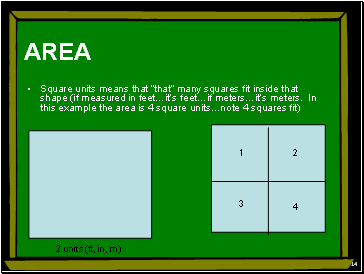14

AREA

Square units means that “that” many squares fit inside that shape (if measured in feet…it’s feet…if meters…it’s meters. In this example the area is 4 square units…note 4 squares fit)

1

2 units (ft, in, m)

2

3

4

Slide 15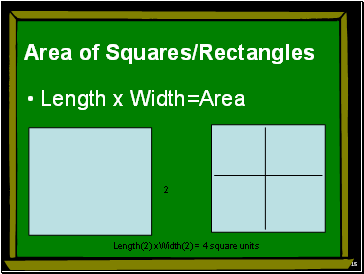15

## Area of Squares/Rectangles

Length x Width=Area

2

Length(2) xWidth(2) = 4 square units

Slide 16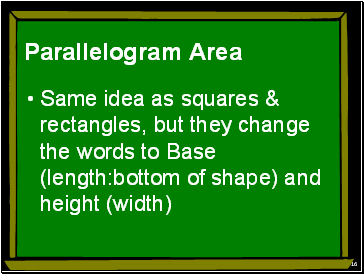16

## Parallelogram Area

Same idea as squares & rectangles, but they change the words to Base (length:bottom of shape) and height (width)

Slide 17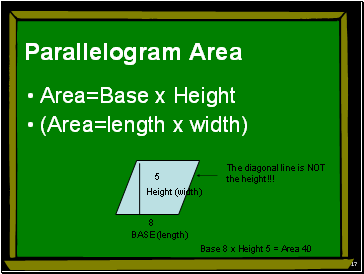17

Parallelogram Area

Area=Base x Height

(Area=length x width)

BASE (length)

Height (width)

8

5

Base 8 x Height 5 = Area 40

The diagonal line is NOT

the height!!!

Slide 18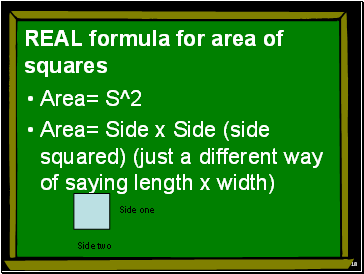18

## Real formula for area of squares

Area= S^2

Area= Side x Side (side squared) (just a different way of saying length x width)

Side one

Side two

Slide 19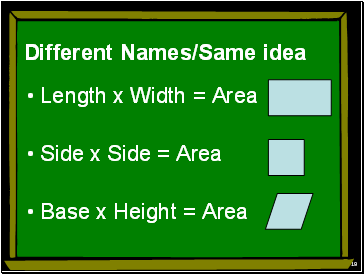19

## Different Names/Same idea

Length x Width = Area

Side x Side = Area

Base x Height = Area

Slide 20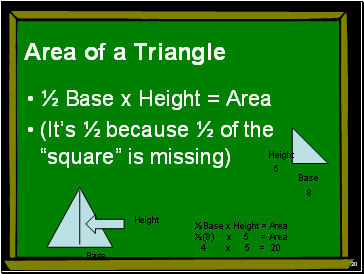20

## Area of a Triangle

½ Base x Height = Area

(It’s ½ because ½ of the “square” is missing)

Base

Height

Height

Base

8

5

½ Base x Height = Area

½ (8) x 5 = Area

4 x 5 = 20

Slide 21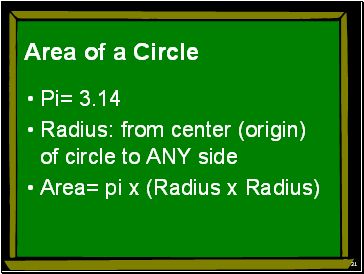21

## Area of a Circle

Pi= 3.14

Radius: from center (origin) of circle to ANY side

Slide 22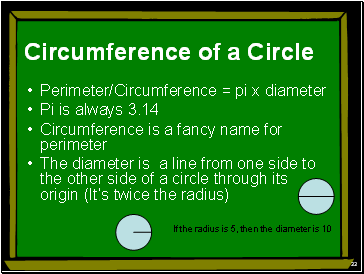22

## Circumference of a Circle

Perimeter/Circumference = pi x diameter

Pi is always 3.14

Circumference is a fancy name for perimeter

The diameter is a line from one side to the other side of a circle through its origin (It’s twice the radius)

If the radius is 5, then the diameter is 10

Slide 23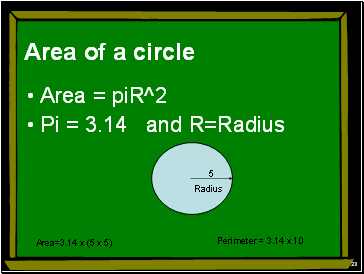23

Area of a circle

Area = piR^2

5

Area=3.14 x (5 x 5)

Perimeter = 3.14 x 10

Go to page:
1  2  3